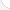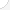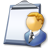Petroleum engineers Description: Devise methods to improve oil and gas well production and determine the need for new or modified tool designs. Oversee drilling and offer technical advice to achieve economical and satisfactory progress.Source: Bureau of Labor Statistics, U.S. Department of Labor, Occupational Outlook Handbook, 2008-09 Edition at http://www.bls.gov/OCO/Complete Job Profile: http://www.bls.gov/oco/ocos027.htmSalary: \$50,001 or more per yearComments:

There are 42 math topics Petroleum engineers need to know.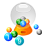Basic Math / Algebra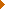FractionsDecimalsRatio and ProportionPercentCustomary MeasurementMetric MeasurementMeasurement ConversionBasic StatisticsPowers and RootsNegative NumbersBasic Problem Solving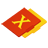First-Year AlgebraUsing FormulasLinear EquationsLinear InequalitiesOperations with PolynomialsFactoring PolynomialsRational ExpressionsCoordinate Graphing 2DLinear SystemsQuadratic EquationsAlgebraic Representation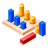GeometryBasic TerminologySimilarityPythagorean TheoremConstructionsAreaVolumeTransformationsMake/Use 3D Drawings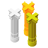Second-Year Algebra / TrigonometryFunctionsVariationLogarithmsCoordinate Graphing 3DAdvanced StatisticsNon-Linear Systems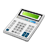Other TopicsCalculus and Higher MathBasic Calculator UseScientific Calculator UseComputer UseGroup Problem SolvingInductive/Deductive ReasoningMathematical Modeling